Question

# Use the Hardy-Weinberg equations (p2 + 2pq + q2 = 1 and p + q =...

Use the Hardy-Weinberg equations (p2 + 2pq + q2 = 1 and p + q = 1) to find the genotypes of the following hypothetical phenotypic information:

a.       Within a particular population, you observe that 450 individuals have freckles (dominant, F) and 550 do not have freckles (recessive, f).

b.      Homozygous dominant (frequency) ______(A)_________

c.       Heterozygous (frequency) ______(B)_____________

d.      Homozygous recessive (frequency) _______(C)____________

Frequency of freckles = q^2= 550/1000= 0.55

Frequency of recessive allele =q= sqrt of 0.55= 0.74

Frequency of dominant allele= p=1- 0.74= 0.26

Frequency of Homozygous dominant= pp= 0.26*0.26= 0.07

Frequency of heterozygote =pq= 2*0.26*0.74= 0.38

Frequency of Homozygous recessive= 0.74*0.74= 0.55

#### Earn Coins

Coins can be redeemed for fabulous gifts.

Similar Homework Help Questions
• ### Equations: p + q=1 p2 + 2(p)(q) + q2 1) In a population with Hardy-Weinberg equilibrium,...

Equations: p + q=1 p2 + 2(p)(q) + q2 1) In a population with Hardy-Weinberg equilibrium, the frequency of a dominant allele for a gene is 85% (0.85) while the frequency of the recessive allele for the same gene is 15% (0.15). 0.0005 0.013 0.675 0.723 0.255 0.50 0.023 2) proportion of individuals will be homozygous recessive? 0.0005 0.013 0.675 0.723 0.255 0.50 0.023 3) proportion of individuals will be homozygous dominant? 0.0005 0.723 0.013 0.675 0.255 0.50 0.023 4)...

• ### Hardy Weinberg assignment P + Q = 1 In which P represents frequency of dominant allele...

Hardy Weinberg assignment P + Q = 1 In which P represents frequency of dominant allele and Q represents frequency of the recessive allele P2 + 2PQ + Q2 =1 P2 represents frequency of homozygous dominant 2PQ represents frequency of heterozygous Q2 represents frequency of homozygous recessive Consider a population of beetles on an island. There are 1000 beetles and they have different colored wings. Black wings are dominant over silver wings. Calculate the allele and the genotypic frequencies in...

• ### 2.3 Problem 3 The Hardy-Weinberg equation is useful for predicting the percent of a hu- man...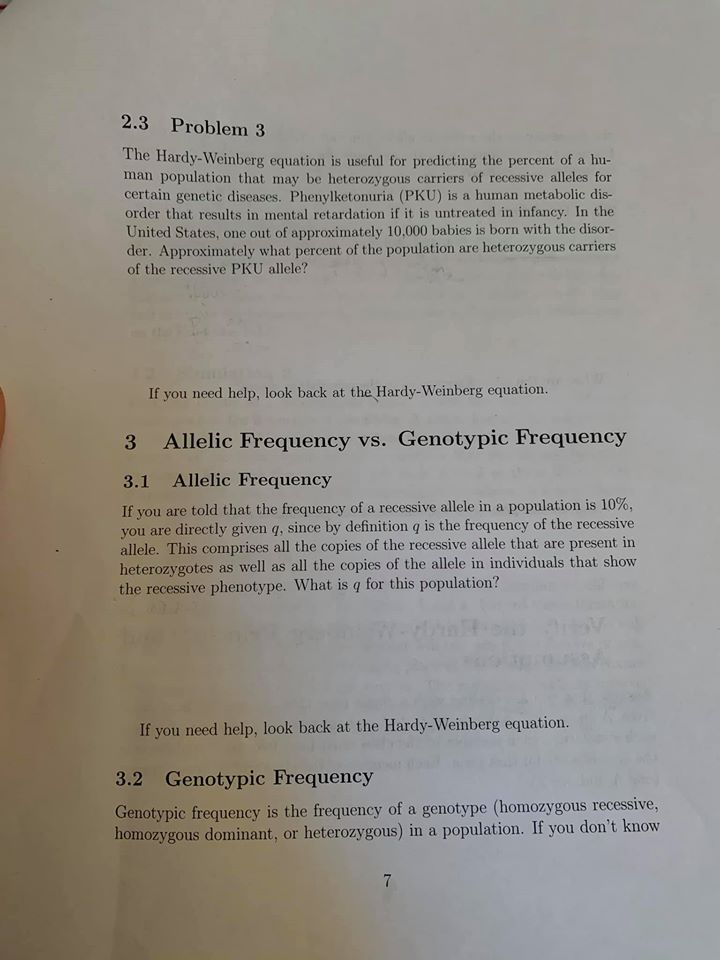2.3 Problem 3 The Hardy-Weinberg equation is useful for predicting the percent of a hu- man population that may be heterozygous carriers of recessive alleles for certain genetic diseases. Phenylketonuria (PKU) is a human metabolic dis- order that results in mental retardation if it is untreated in infancy. In the United States, one out of approximately 10.000 babies is born with the disor- der. Approximately what percent of the population are heterozygous carriers of the recessive PKU allele? If you...

• ### The Hardy-Weinberg principle and its equations predict that frequencies of alleles and genotypes remain constant from generation to generation in populations that are not evolving

.1. The Hardy-Weinberg principle and its equations predict that frequencies of alleles and genotypes remain constant from generation to generation in populations that are not evolving. What five conditions does this prediction assume to be true about such a population? a._______  b._______  c._______  d._______  e._______  2. Before beginning the activity, answer the following general Hardy-Weinberg problems for practice (assume that the population is at Hardy-Weinberg equilibrium).a. If the frequency of a recessive allele is 0.3, what is the frequency of the dominant...

• ### please click on the photo to see all of it The basic equations of Hardy-Weinberg Equilibrium...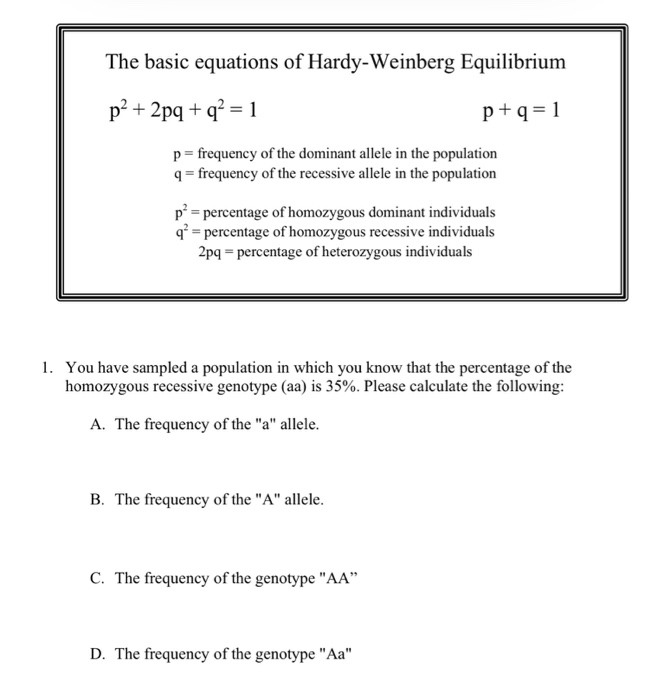please click on the photo to see all of it The basic equations of Hardy-Weinberg Equilibrium p² + 2pq + q2 = 1 p+q=1 p= frequency of the dominant allele in the population 9 = frequency of the recessive allele in the population př= percentage of homozygous dominant individuals q* = percentage of homozygous recessive individuals 2pq - percentage of heterozygous individuals 1. You have sampled a population in which you know that the percentage of the homozygous recessive genotype...

• ### 1. According to the Hardy-Weinberg theorem, p + q = 1 and p2 + 2pq +q2...

1. According to the Hardy-Weinberg theorem, p + q = 1 and p2 + 2pq +q2 = 1. What does each of these formulas mean (how are they derived)?

• ### EXERCISE 6 HARDY-WEINBERG EQUILIBRIUM Work in a small group or alone to complete this exercise. In...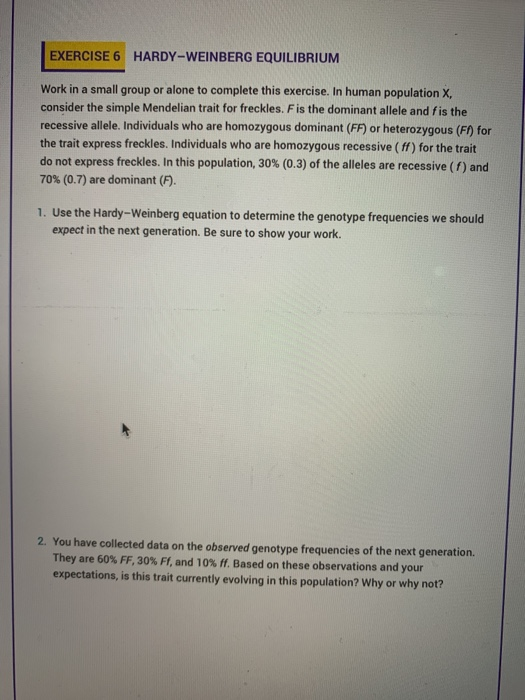EXERCISE 6 HARDY-WEINBERG EQUILIBRIUM Work in a small group or alone to complete this exercise. In human population X consider the simple Mendelian trait for freckles. F is the dominant allele and fis the recessive allele. Individuals who are homozygous dominant (FF) or heterozygous (F) for the trait express freckles. Individuals who are homozygous recessive (ff) for the trait do not express freckles. In this population, 30% (0.3) of the alleles are recessive (1) and 70% (0.7) are dominant (F)....

• ### reting Data: Hardy-Weinberg Equation 2 of 10 you use the Hardy Weinberg equation to answer questions...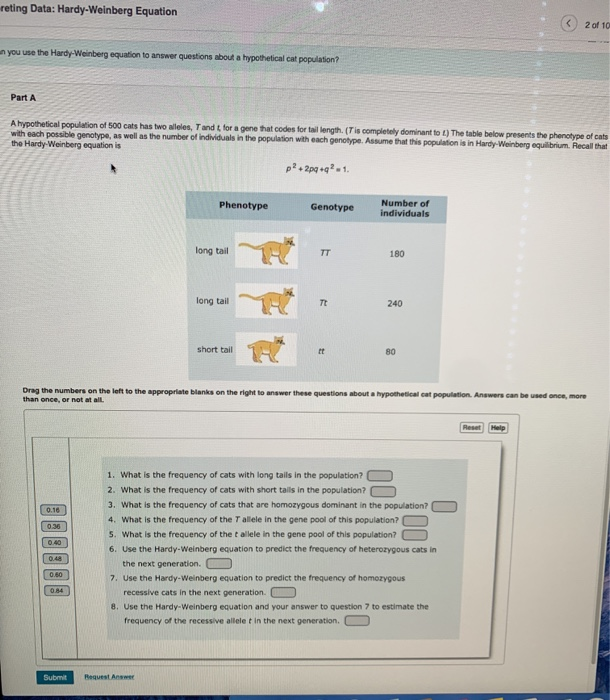reting Data: Hardy-Weinberg Equation 2 of 10 you use the Hardy Weinberg equation to answer questions about a hypotheticalcat population Part A A hypothetical population of 500 cats has two wees, Tandt for a gene that codes for tail length (Tis completely dominantot) The table below presents the phenotype of cats with each possible genotype, as well as the number of individuals in the population with each genotype. Assume that this population is in Hardy-Weinberg equilibrium Recall that the Hardy...

• ### Hardy-Weinberg Practice Problems: You need to list equations used and provide steps of problem solving. Providing...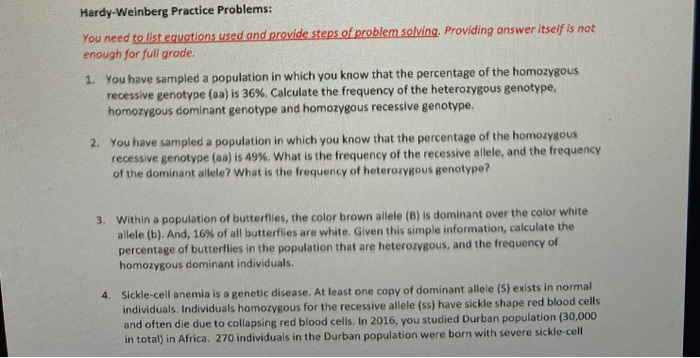Hardy-Weinberg Practice Problems: You need to list equations used and provide steps of problem solving. Providing answer itself is not enough for full grade. 1. You have sampled a population in which you know that the percentage of the homozygous recessive genotype (aa) is 36%. Calculate the frequency of the heterozygous genotype, homozygous dominant genotype and homozygous recessive genotype. 2. You have sampled a population in which you know that the percentage of the homozygous recessive genotype (aa) is 49%....

• ### Question 13 2 pts The Hardy-Weinberg equation is p+2pq+q?-1. In a case where one allele represented...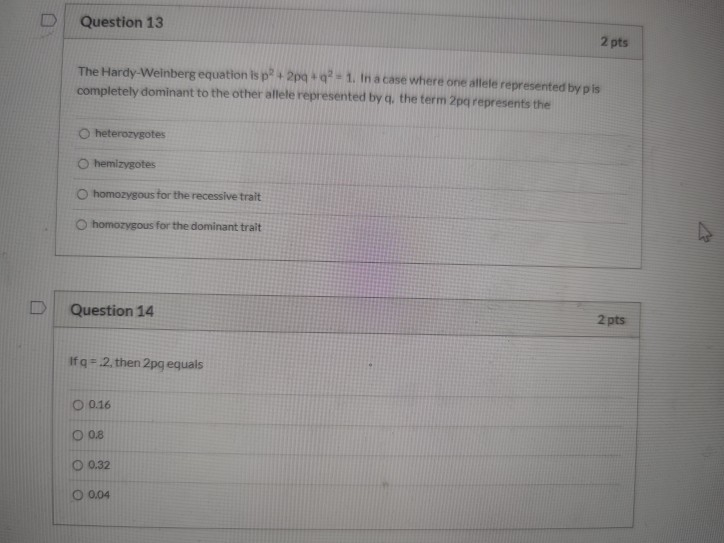Question 13 2 pts The Hardy-Weinberg equation is p+2pq+q?-1. In a case where one allele represented by pis completely dominant to the other allele represented by q, the term 2pa represents the heterozygotes hemizygotes homozygous for the recessive trait homozygous for the dominant trait Question 14 2 pts If q=2. then 2pg equals 0.16 O 0.8 O 0.32 0 0.04